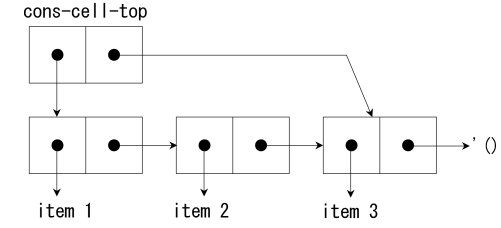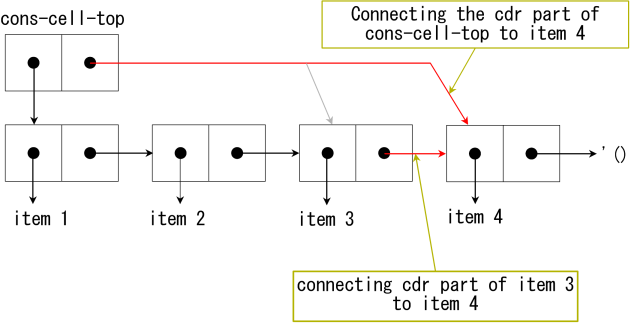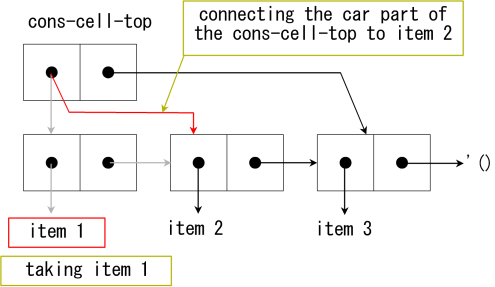# 10. Assignment

## 1. Introduction

As Scheme is a functional programming language, you can write programs without assignment in principle. However, some algorithm can be implemented easily by using assignment. Especially internal states and continuations require assignment.

Even assignment is convenient and easy to understand, it has substantial drawback. See SICP: 3.1 Assignment and Local State and Why Functional Programming Matters.

Forms for assignment defined in R5RS are set!, set-car!, set-cdr!, string-set!, vector-set! and etc. In addition there are several implementation depending assignment forms. As assignment changes values of parameters, it is called destructive. In Scheme, names of destructive operators end with ! to warn programmers.

## 2. set!

The set! is used to assign a parameter. The operator can not assign a value to a S-expression which is different from the setf of the Common Lisp. Parameters should be defined before assignment.
```(define var 1)
(set! var (* var 10))
var ⇒ 10

(let ((i 1))
(set! i (+ i 3))
i)
⇒ 4
```

## 3. Assignment and Internal status

### 3.1. Static Scope (Lexical Closure)

The scope of variables in Scheme is in the parentheses in that the variables are defined on the source code. The scope as written in the source code is called lexical closure or static scope. This way of scope eliminates bags, as you can grasp the scope of parameters quite easily — written on the source code. On the other hand, there is another way of determining scopes, called dynamic scope. In this way the scope is determined when the program is executed. This way is not used nowadays often because of difficulties of bug fixing.

The let, lambda, and letrec form make closures. The arguments of lambda expression are valid in the function definition. As let is a syntax sugar for lambda, the same thing happens.

### 3.2. Implementation of Internal States using the Lexical Closure and Assignment

You can make procedures with internal status by using the lexical closure. For instance, procedure that simulate bank accounts can be written like as follows:
Ten dollars should be donated to make new bank account. The function takes one integer argument. The positive values represent donation and the negative withdrawing. Negative balance is allowed for simplification.
```(define bank-account
(let ((balance 10))
(lambda (n)
(set! balance (+ balance n))
balance)))
```
The procedure assigns (+ balance n) to the balance.
Following is the result of calling this function.
```(bank-account 20)     ; donating 20 dollars
;Value: 30

(bank-account -25)     ; withdrawing 25 dollars
;Value: 5
```

As you can write procedures that returns a procedure using Scheme, you can write a function that create 'bank account'.
This example implies that it is easy to make an object oriented (OO) language using functional programming language. In fact, only few more is required from here to make an OO language.

```(define (make-bank-account balance)
(lambda (n)
(set! balance (+ balance n))
balance))
```
```(define gates-bank-account (make-bank-account 10))   ; Gates makes a bank account by donating  10 dollars
;Value: gates-bank-account

(gates-bank-account 50)                              ; donating 50 dollars
;Value: 60

(gates-bank-account -55)                             ; withdrawing 55 dollars
;Value: 5

(define torvalds-bank-account (make-bank-account 100))  ; Torvalds makes a bank account by donating 100 dollars
;Value: torvalds-bank-account

(torvalds-bank-account -70)                             ; withdrawing 70 dollars
;Value: 30

(torvalds-bank-account 300)                             ; donating 300 dollars
;Value: 330
```

### 3.3. Side effect

The main goal of Scheme procedure is to return a value and other effect is called side effect. Assignment and IO are side effects.

### Exercise 1

Modify make-bank-account so that withdrawing more than balance causes error.
hint: Use begin to group more than one S-expressions.

## 4. Destructive Operations on Lists (set-car!, set-cdr!)

The set-car! and set-cdr! assign a value to the car and cdr part of a cons cell, respectively. These operators can assign a value to a S-expression, which is different in the case of set!.
```(define tree '((1 2) (3 4 5) (6 7 8 9)))

(set-car! (car tree) 100)  ; changing 1 to 100

tree
((100 2) (3 4 5) (6 7 8 9))

(set-cdr! (third tree) '(a b c)) ; changing  '(7 8 9) to '(a b c)

tree
⇒ ((100 2) (3 4 5) (6 a b c))
```

### 4.1. Queue

Queue can be implemented by using set-car! and set-cdr!. Queue is a first in first out (FIFO) date structure while ordinary list is first in last out (FILO). Figure 1 shows the data structure of queue. The pointer of the car part of cons-cell-top points to the list and that of the cdr part to the last cons cell of the list.Figure 1: The data structure of queue.

Enqueue is performed with following procedure (Fig. 2):

1. Redirecting the cdr part of the last cons cell which is accessible directly by (cdr cons-cell-top) to a new item.
2. Redirecting the cdr part of cons-cell-top to the new item.Figure 2: The procedure of adding 'item 4' to the queue.

Dequeue is performed with following procedure (Fig. 3)

1. Storing the top item of the list to a local variable.
2. Redirecting the car part of the cons-cell-top to the second item of the list.Figure 3: The procedure of popping 'item 1' from the queue.

[code 1] shows a implementation of queue. The function enqueue! returns a queue in that the obj is added at the last of the queue. The function dequeue! removes the first item from the queue and return the first item.

[code 1]

```(define (make-queue)
(cons '() '()))

(define (enqueue! queue obj)
(let ((lobj (cons obj '())))
(if (null? (car queue))
(begin
(set-car! queue lobj)
(set-cdr! queue lobj))
(begin
(set-cdr! (cdr queue) lobj)
(set-cdr! queue lobj)))
(car queue)))

(define (dequeue! queue)
(let ((obj (car (car queue))))
(set-car! queue (cdr (car queue)))
obj))
```
```(define q (make-queue))
;Value: q

(enqueue! q 'a)
;Value 12: (a)

(enqueue! q 'b)
;Value 12: (a b)

(enqueue! q 'c)
;Value 12: (a b c)

(dequeue! q)
;Value: a

q
;Value 13: ((b c) c)
```

## 5. Summary

I have explained about assignment and scope of variables in this chapter. Even assignment is not used in Scheme often, it is indispensable for some algorithm and data structure.

Too much use of assignment makes your code ugly. Use assignment only it is really required with care.

I will explain about data structures used in Scheme in following several chapters.

```(define (make-bank-account amount)